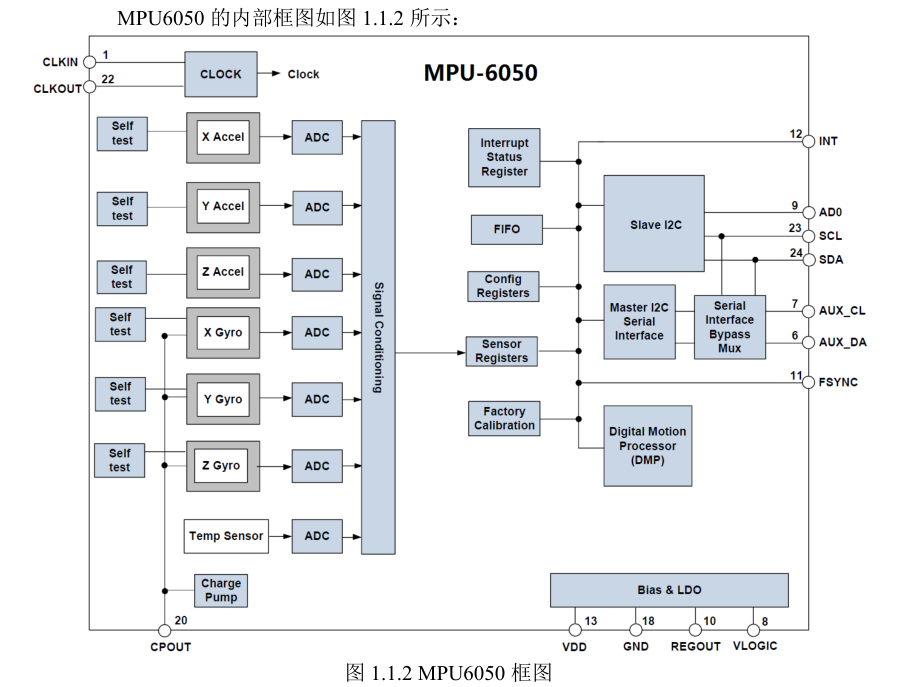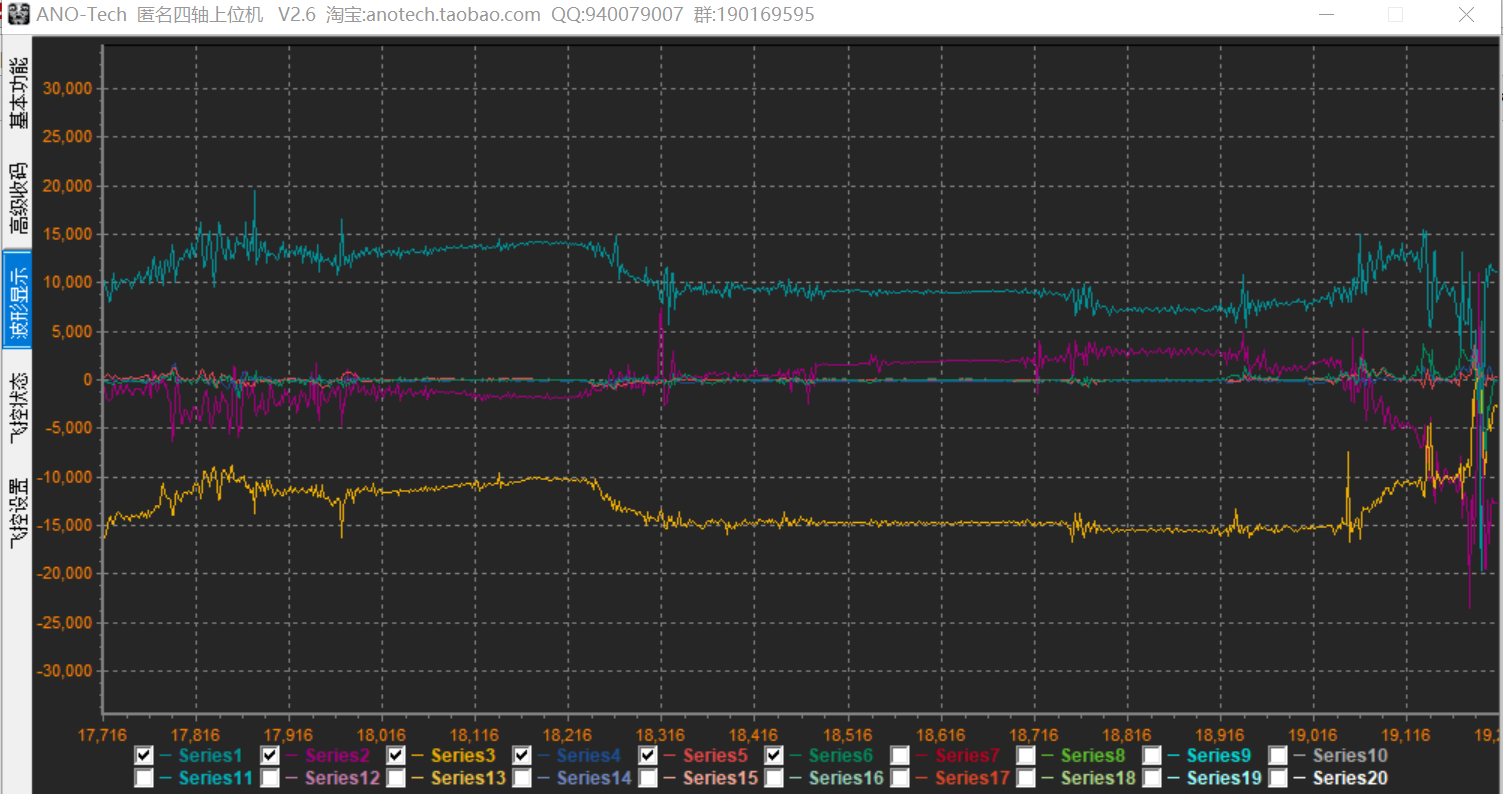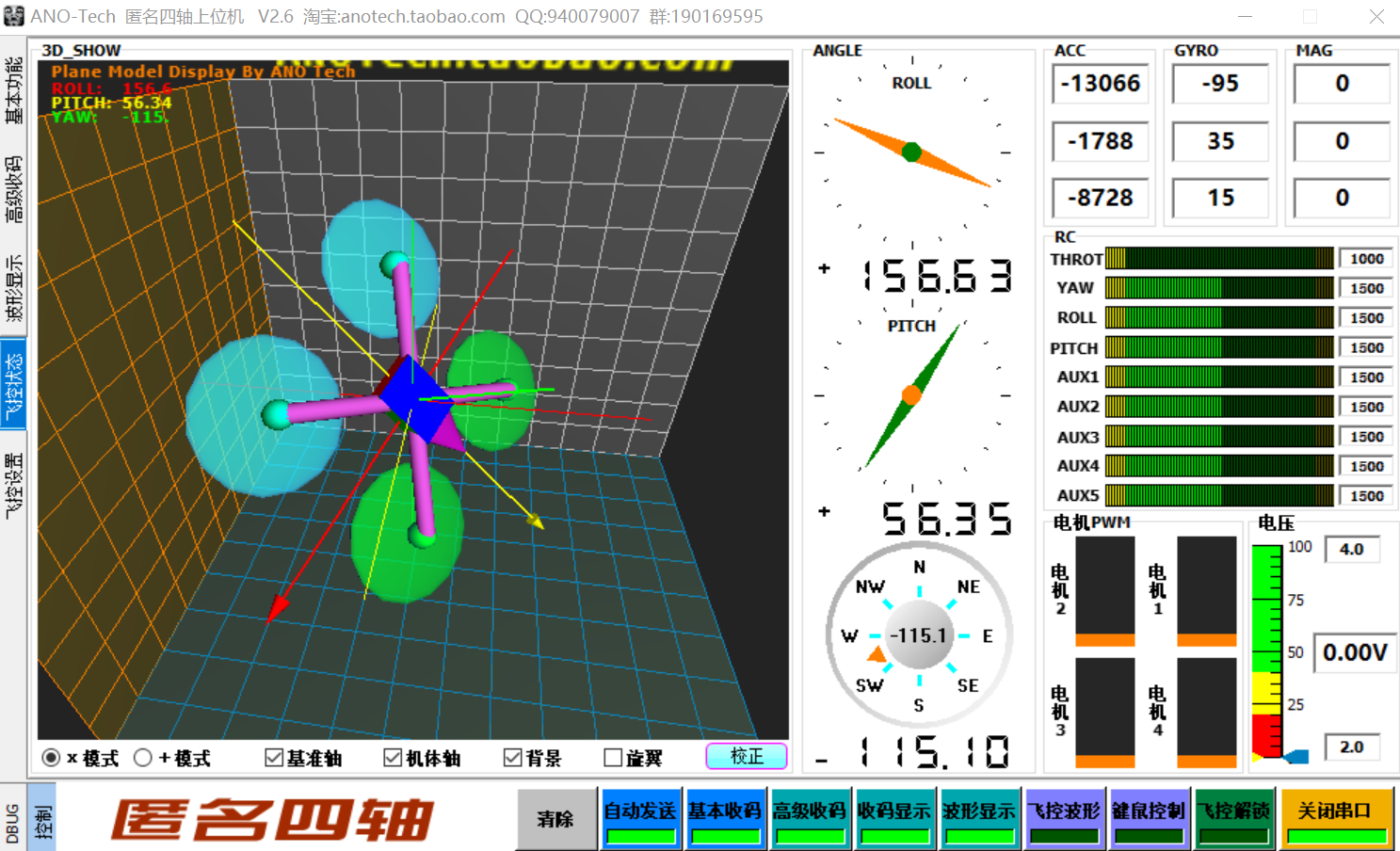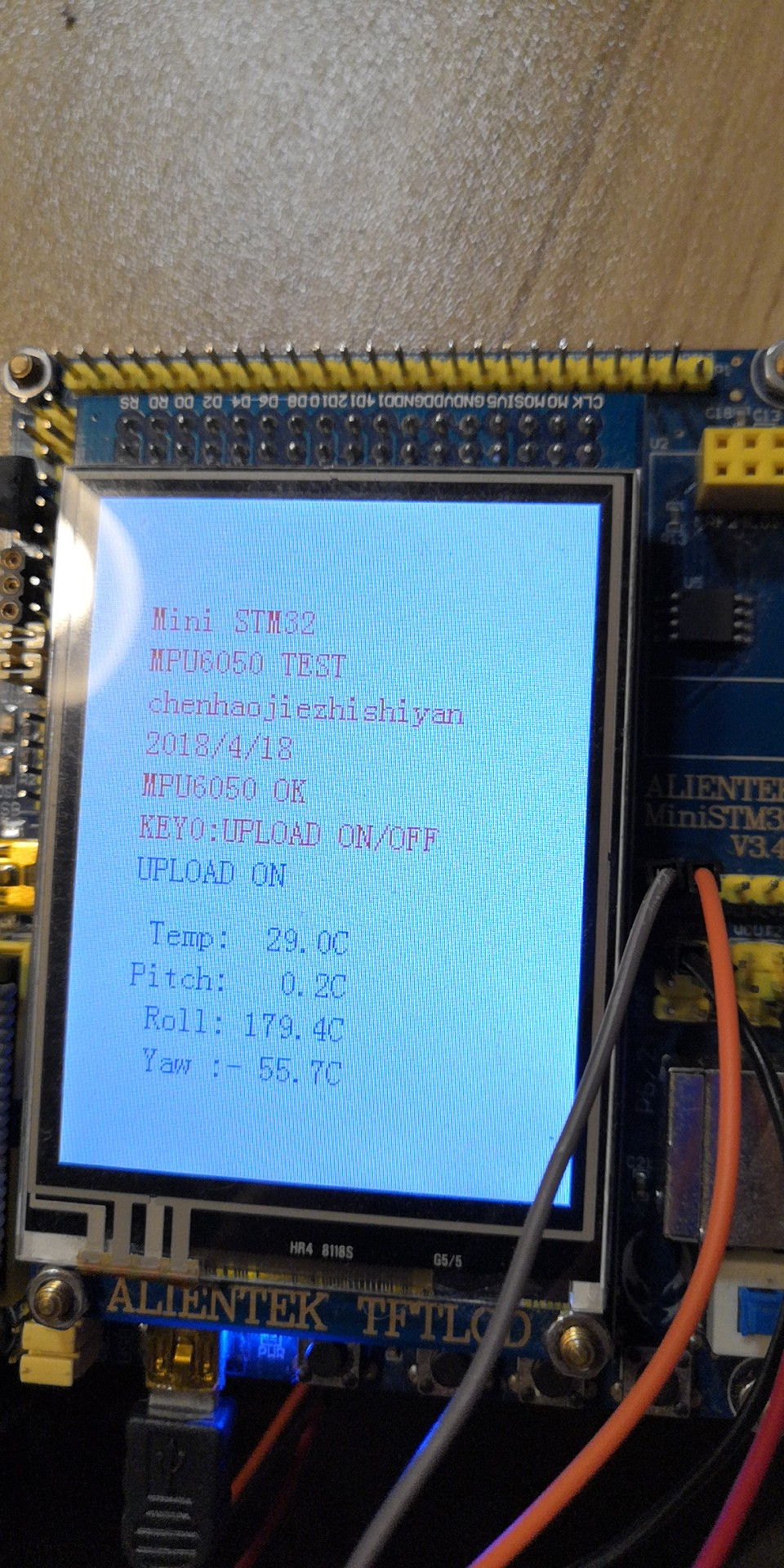# STM32F103之实验6 采用MPU6050及DMP解算移动机器人姿态实验1 ）初始化 IIC  接口
MPU6050 采用 IIC 与 STM32F1 通信，所以我们需要先初始化与 MPU6050 连接的 SDA和 SCL 数据线。
2 ）复位 MPU6050

3 ）设置角速度传感器（陀螺仪）和加速度传感器的满量程范围

4 ）设置其他参数

5 ）配置系统时钟源并使能角速度传感器和加速度传感器

至此，MPU6050 的初始化就完成了，可以正常工作了（其他未设置的寄存器全部采用默认值即可），接下来，我们就可以读取相关寄存器，得到加速度传感器、角速度传感器和温度传感器的数据了。

//初始化MPU6050

#include "mpu6050.h"
#include "sys.h"
#include "delay.h"
#include "usart.h"
//初始化MPU6050
//返回值:0,成功
//    其他,错误代码
u8 MPU_Init(void)

u8 res;
MPU_IIC_Init();//初始化IIC总线
MPU_Write_Byte(MPU_PWR_MGMT1_REG,0X80);    //复位MPU6050
delay_ms(100);
MPU_Write_Byte(MPU_PWR_MGMT1_REG,0X00);    //唤醒MPU6050
MPU_Set_Gyro_Fsr(3);                    //陀螺仪传感器,±2000dps
MPU_Set_Accel_Fsr(0);                    //加速度传感器,±2g
MPU_Set_Rate(50);                        //设置采样率50Hz
MPU_Write_Byte(MPU_INT_EN_REG,0X00);    //关闭所有中断
MPU_Write_Byte(MPU_USER_CTRL_REG,0X00);    //I2C主模式关闭
MPU_Write_Byte(MPU_FIFO_EN_REG,0X00);    //关闭FIFO
MPU_Write_Byte(MPU_INTBP_CFG_REG,0X80);    //INT引脚低电平有效
{
MPU_Write_Byte(MPU_PWR_MGMT1_REG,0X01);    //设置CLKSEL,PLL X轴为参考
MPU_Write_Byte(MPU_PWR_MGMT2_REG,0X00);    //加速度与陀螺仪都工作
MPU_Set_Rate(50);                        //设置采样率为50Hz
}else return 1;
return 0;
}

//设置MPU6050陀螺仪传感器满量程范围
//fsr:0,±250dps;1,±500dps;2,±1000dps;3,±2000dps
//返回值:0,设置成功
//    其他,设置失败
u8 MPU_Set_Gyro_Fsr(u8 fsr)
{
return MPU_Write_Byte(MPU_GYRO_CFG_REG,fsr<<3);//设置陀螺仪满量程范围
}

//设置MPU6050加速度传感器满量程范围
//fsr:0,±2g;1,±4g;2,±8g;3,±16g
//返回值:0,设置成功
//    其他,设置失败
u8 MPU_Set_Accel_Fsr(u8 fsr)
{
return MPU_Write_Byte(MPU_ACCEL_CFG_REG,fsr<<3);//设置加速度传感器满量程范围
}

//设置MPU6050的数字低通滤波器
//lpf:数字低通滤波频率(Hz)
//返回值:0,设置成功
//    其他,设置失败
u8 MPU_Set_LPF(u16 lpf)
{
u8 data=0;
if(lpf>=188)data=1;
else if(lpf>=98)data=2;
else if(lpf>=42)data=3;
else if(lpf>=20)data=4;
else if(lpf>=10)data=5;
else data=6;
return MPU_Write_Byte(MPU_CFG_REG,data);//设置数字低通滤波器
}

//设置MPU6050的采样率(假定Fs=1KHz)
//rate:4~1000(Hz)
//返回值:0,设置成功
//    其他,设置失败
u8 MPU_Set_Rate(u16 rate)//设置MPU6050的采样率(假定Fs=1KHz)
{
u8 data;
if(rate>1000)rate=1000;
if(rate<4)rate=4;
data=1000/rate-1;
data=MPU_Write_Byte(MPU_SAMPLE_RATE_REG,data);    //设置数字低通滤波器
return MPU_Set_LPF(rate/2);    //自动设置LPF为采样率的一半
}
//得到陀螺仪值(原始值)
//gx,gy,gz:陀螺仪x,y,z轴的原始读数(带符号)
//返回值:0,成功
//    其他,错误代码
u8 MPU_Get_Gyroscope(short *gx,short *gy,short *gz)/得到陀螺仪值(原始值)
{
u8 buf,res;
if(res==0)
{
*gx=((u16)buf<<8)|buf;
*gy=((u16)buf<<8)|buf;
*gz=((u16)buf<<8)|buf;
}
return res;;
}
//得到加速度值(原始值)
//gx,gy,gz:陀螺仪x,y,z轴的原始读数(带符号)
//返回值:0,成功
//    其他,错误代码
u8 MPU_Get_Accelerometer(short *ax,short *ay,short *az)//得到加速度值(原始值)
{
u8 buf,res;
if(res==0)
{
*ax=((u16)buf<<8)|buf;
*ay=((u16)buf<<8)|buf;
*az=((u16)buf<<8)|buf;
}
return res;;
}
//IIC连续写
//reg:寄存器地址
//len:写入长度
//buf:数据区
//返回值:0,正常
//    其他,错误代码
u8 MPU_Write_Len(u8 addr,u8 reg,u8 len,u8 *buf)
{
u8 i;
MPU_IIC_Start();
if(MPU_IIC_Wait_Ack())    //等待应答
{
MPU_IIC_Stop();
return 1;
}
MPU_IIC_Send_Byte(reg);    //写寄存器地址
MPU_IIC_Wait_Ack();        //等待应答
for(i=0;i<len;i++)
{
MPU_IIC_Send_Byte(buf[i]);    //发送数据
if(MPU_IIC_Wait_Ack())        //等待ACK
{
MPU_IIC_Stop();
return 1;
}
}
MPU_IIC_Stop();
return 0;

u8 MPU_Write_Byte(u8 reg,u8 data)     //IIC写一个字节

//mpu6050dmp的初始化函数。
//返回值:0,正常
//    其他,失败
u8 mpu_dmp_init(void)
{
u8 res=0;
MPU_IIC_Init();     //初始化IIC总线
if(mpu_init()==0)    //初始化MPU6050
{
res=mpu_set_sensors(INV_XYZ_GYRO|INV_XYZ_ACCEL);//设置所需要的传感器
if(res)return 1;
res=mpu_configure_fifo(INV_XYZ_GYRO|INV_XYZ_ACCEL);//设置FIFO
if(res)return 2;
res=mpu_set_sample_rate(DEFAULT_MPU_HZ);    //设置采样率
if(res)return 3;
if(res)return 4;
res=dmp_set_orientation(inv_orientation_matrix_to_scalar(gyro_orientation));//设置陀螺仪方向
if(res)return 5;
res=dmp_enable_feature(DMP_FEATURE_6X_LP_QUAT|DMP_FEATURE_TAP|    //设置dmp功能
DMP_FEATURE_ANDROID_ORIENT|DMP_FEATURE_SEND_RAW_ACCEL|DMP_FEATURE_SEND_CAL_GYRO|
DMP_FEATURE_GYRO_CAL);
if(res)return 6;
res=dmp_set_fifo_rate(DEFAULT_MPU_HZ);    //设置DMP输出速率(最大不超过200Hz)
if(res)return 7;
res=run_self_test();        //自检
if(res)return 8;
res=mpu_set_dmp_state(1);    //使能DMP
if(res)return 9;
}else return 10;
return 0;
}

mpu_init 函数，初始化 MPU6050，之后就是设置 DMP 所用传感器、FIFO、采样率和加载

q0=quat / q30; //q30 格式转换为浮点数
q1=quat / q30;
q2=quat / q30;
q3=quat / q30;
//计算得到俯仰角/横滚角/航向角pitch=asin(-2 * q1 * q3 + 2 * q0* q2)* 57.3; //俯仰角
roll=atan2(2 * q2 * q3 + 2 * q0 * q1, -2 * q1 * q1 - 2 * q2* q2 + 1)* 57.3; //横滚角
yaw=atan2(2*(q1*q2 + q0*q3),q0*q0+q1*q1-q2*q2-q3*q3) * 57.3; //航向角

quat~ quat是 MPU6050 的 DMP 解算后的四元数，q30 格式，所以要除以一个
2 的 30 次方，其中 q30 是一个常量：1073741824，即 2 的 30 次方，然后带入公式，计算出

//pitch:俯仰角 精度:0.1°   范围:-90.0° <---> +90.0°
//roll:横滚角  精度:0.1°   范围:-180.0°<---> +180.0°
//yaw:航向角   精度:0.1°   范围:-180.0°<---> +180.0°
//返回值:0,正常
//    其他,失败

u8 mpu_dmp_get_data(float *pitch,float *roll,float *yaw)
{
float q0=1.0f,q1=0.0f,q2=0.0f,q3=0.0f;
unsigned long sensor_timestamp;
short gyro, accel, sensors;
unsigned char more;
long quat;
if(dmp_read_fifo(gyro, accel, quat, &sensor_timestamp, &sensors,&more))return 1;
if (sensors & INV_XYZ_GYRO )
send_packet(PACKET_TYPE_GYRO, gyro);
if (sensors & INV_XYZ_ACCEL)
send_packet(PACKET_TYPE_ACCEL, accel);
if(sensors&INV_WXYZ_QUAT)
{
q0 = quat / q30;    //q30格式转换为浮点数
q1 = quat / q30;
q2 = quat / q30;
q3 = quat / q30;
//计算得到俯仰角/横滚角/航向角
*pitch = asin(-2 * q1 * q3 + 2 * q0* q2)* 57.3;    // pitch
*roll  = atan2(2 * q2 * q3 + 2 * q0 * q1, -2 * q1 * q1 - 2 * q2* q2 + 1)* 57.3;    // roll
*yaw   = atan2(2*(q1*q2 + q0*q3),q0*q0+q1*q1-q2*q2-q3*q3) * 57.3;    //yaw
}else return 2;
return 0;
}

int main(void)
{
u8 t=0,report=1;            //默认开启上报
u8 key;
float pitch,roll,yaw;         //欧拉角
short aacx,aacy,aacz;        //加速度传感器原始数据
short gyrox,gyroy,gyroz;    //陀螺仪原始数据
short temp;                    //温度

NVIC_PriorityGroupConfig(NVIC_PriorityGroup_2);
delay_init();             //延时函数初始化
uart_init(500000);         //串口初始化为500 000
usmart_dev.init(72);        //初始化USMART
LED_Init();                      //初始化与LED连接的硬件接口
KEY_Init();                    //初始化按键
LCD_Init();                       //初始化LCD
MPU_Init();                    //初始化MPU6050
POINT_COLOR=RED;            //设置字体为红色
LCD_ShowString(30,50,200,16,16,"Mini STM32");
LCD_ShowString(30,70,200,16,16,"MPU6050 TEST");
LCD_ShowString(30,90,200,16,16,"chenhaojiezhishiyan");
LCD_ShowString(30,110,200,16,16,"2018/4/18");
while(mpu_dmp_init())
{
LCD_ShowString(30,130,200,16,16,"MPU6050 Error");
delay_ms(200);
LCD_Fill(30,130,239,130+16,WHITE);
delay_ms(200);
}
LCD_ShowString(30,130,200,16,16,"MPU6050 OK");
POINT_COLOR=BLUE;//设置字体为蓝色
LCD_ShowString(30,200,200,16,16," Temp:    . C");
LCD_ShowString(30,220,200,16,16,"Pitch:    . C");
LCD_ShowString(30,240,200,16,16," Roll:    . C");
LCD_ShowString(30,260,200,16,16," Yaw :    . C");
while(1)
{
key=KEY_Scan(0);
if(key==KEY0_PRES)
{
report=!report;
}
if(mpu_dmp_get_data(&pitch,&roll,&yaw)==0)
{
temp=MPU_Get_Temperature();    //得到温度值
MPU_Get_Accelerometer(&aacx,&aacy,&aacz);    //得到加速度传感器原始数据

MPU_Get_Gyroscope(&gyrox,&gyroy,&gyroz);    //得到陀螺仪数据

if(report)mpu6050_send_data(aacx,aacy,aacz,gyrox,gyroy,gyroz);//用自定义帧发送加速度和陀螺仪原始数据

if(report)usart1_report_imu(aacx,aacy,aacz,gyrox,gyroy,gyroz,(int)(roll*100),(int)(pitch*100),(int)(yaw*10));

if((t%10)==0)
{
if(temp<0)
{
LCD_ShowChar(30+48,200,'-',16,0);        //显示负号
temp=-temp;        //转为正数
}else LCD_ShowChar(30+48,200,' ',16,0);        //去掉负号
LCD_ShowNum(30+48+8,200,temp/100,3,16);        //显示整数部分
LCD_ShowNum(30+48+40,200,temp%10,1,16);        //显示小数部分
temp=pitch*10;
if(temp<0)
{
LCD_ShowChar(30+48,220,'-',16,0);        //显示负号
temp=-temp;        //转为正数
}else LCD_ShowChar(30+48,220,' ',16,0);        //去掉负号
LCD_ShowNum(30+48+8,220,temp/10,3,16);        //显示整数部分
LCD_ShowNum(30+48+40,220,temp%10,1,16);        //显示小数部分
temp=roll*10;
if(temp<0)
{
LCD_ShowChar(30+48,240,'-',16,0);        //显示负号
temp=-temp;        //转为正数
}else LCD_ShowChar(30+48,240,' ',16,0);        //去掉负号
LCD_ShowNum(30+48+8,240,temp/10,3,16);        //显示整数部分
LCD_ShowNum(30+48+40,240,temp%10,1,16);        //显示小数部分
temp=yaw*10;
if(temp<0)
{
LCD_ShowChar(30+48,260,'-',16,0);        //显示负号
temp=-temp;        //转为正数
}else LCD_ShowChar(30+48,260,' ',16,0);        //去掉负号
LCD_ShowNum(30+48+8,260,temp/10,3,16);        //显示整数部分
LCD_ShowNum(30+48+40,260,temp%10,1,16);        //显示小数部分
t=0;
LED0=!LED0;//LED闪烁
}
}
t++;
}
}12-212万+

#### 详解MPU6050，用STM32读取原始数据，并相互融合算出俯仰角、翻滚角、偏航角03-242万+

#### 关于MPU6050姿态解算的理解与认识

08-151151

#### （转）四轴飞行器6050六轴传感器软件姿态解算

06-021830

#### 硬件姿态解算

11-087101

#### 模块介绍之六轴陀螺仪MPU6050篇（STM32基本使用）

03-174026

#### 使用STM32 CUBE搭建工程实现MPU6050的角度测量

09-261万+

#### STM32驱动MPU6050（二）——软件实现

07-152万+

#### 陀螺仪mpu6050的调试与dmp库的移植经历©️2020 CSDN 皮肤主题: 技术黑板 设计师: CSDN官方博客点击重新获取扫码支付1.余额是钱包充值的虚拟货币，按照1:1的比例进行支付金额的抵扣。
2.余额无法直接购买下载，可以购买VIP、C币套餐、付费专栏及课程。余额充值